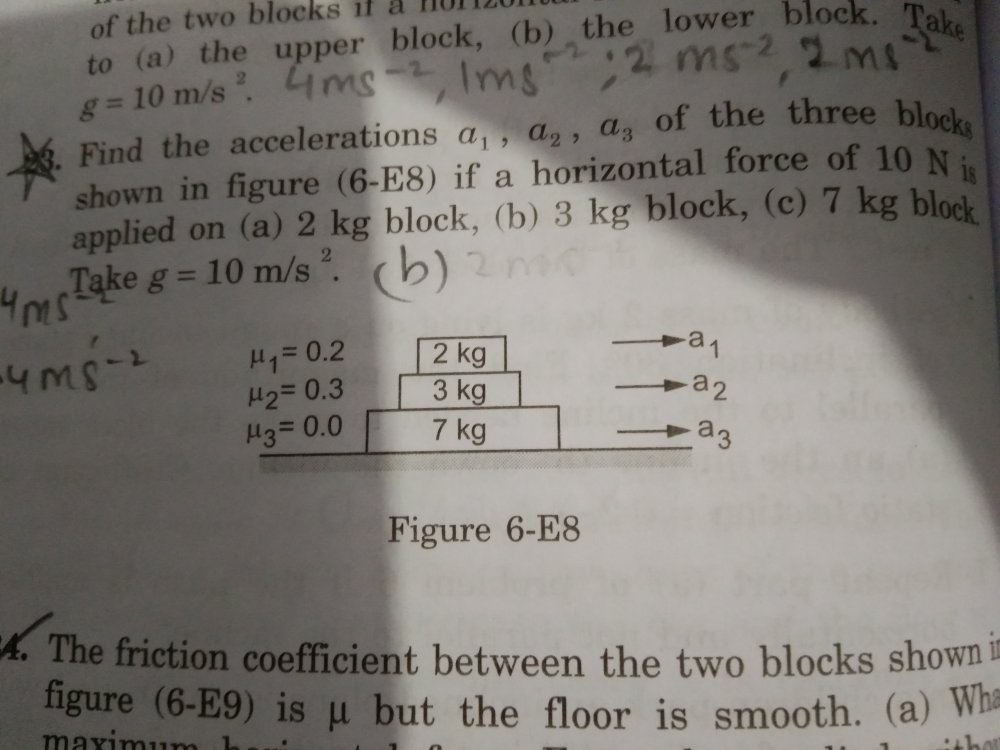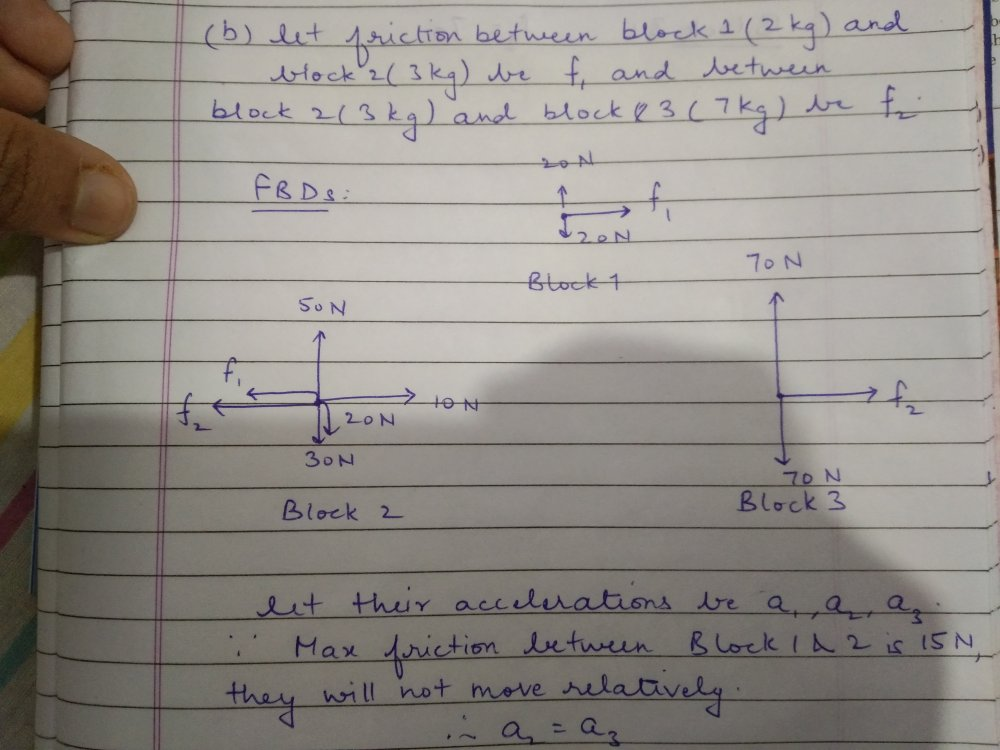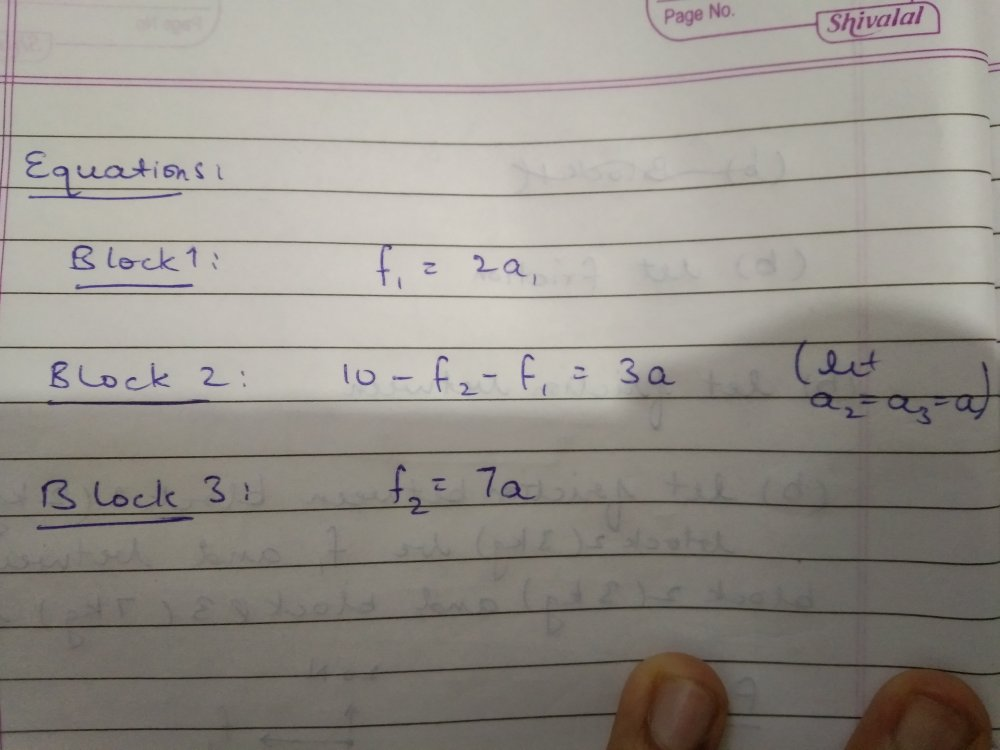Friction

Recommended Posts

How do I do the part b and c of this question (23)? Any hints?

One part of me says that in part b, the max friction between the 3 kg and 7 kg block is 15 N, so there will be no relative motion between them. What about 2kg and 3 kg block?Share on other sites

2 hours ago, Arnav said:

One part of me says that in part b, the max friction between the 3 kg and 7 kg block is 15 N, so there will be no relative motion between them. What about 2kg and 3 kg block?

This question contains enough to be analized.

What have you actually done towards it?

Share on other sites

I tried to, but i could not come to the conclusion whether the friction between block b and c will be able to restrict their relative motion or not

Share on other sites

3 hours ago, Arnav said:

I tried to, but i could not come to the conclusion whether the friction between block b and c will be able to restrict their relative motion or not

I asked what you have done, not what you have not done !Have you assigned all the frictional forces between the blocks and the table and the blocks ?

That will give you a lot of equations and is where you should start.

In other words, write down all the equations of friction.

Edited by studiot
Share on other sites

Here is what I have done so farI am getting 3 equations and 4 variables.  When i see a solution of this question, it is said that blocks 1 and 2 will not move relative to each other as well. I dont get how i can conclude that f₁ and f₂ will be such that all three blocks are not in relative motion. Do i take into account the self adjustable nature of friction? or am i missing something else?

Share on other sites

One suggestion: use variables and substitute the values at the end. In the long run this will make solutions easier.

What is the frictional force between block 1 and block 2?

Share on other sites

Thank you, I see that you are working along the right lines but trying to take short cuts.

Perhaps this is why you have reached the astounding conclusion that the accelerations are different yet the blocks do not move relative to each other.

This is pure Physics speaking.

How can two objects, both starting from zero velocity not have different subsequent velocities for all time if their accelerations are different ?

OK back to the Mathematics.

You have correctly matched some frictional forces to some frictional coefficients.

What about μ3 and F3 ?

The three equations of friction I referred to are

F3 = μ3N3 = 0*12g = 0 Newtons  ,  where N is the normal reaction between the bottom (7kg block) and the table.

F2 = μ2N2 =  ?

F1 = μ1N1 =  ?

I will leave you to complete these and confirm you understand where they come from.

Then you will have all the forces acting on each of three blocks.

For each of those three blocks you have to consider what happens when the 10N force is applied at three different locations.

That is a total of 9 possibilities.

Each of these 9 possibilities can be dealt with by considering the net force (not equilibrium) acting on each block as a free body.

So for the first one apply the 10N to the 7kg block we have

10N acting to the right as shown in the original diagram

and F3 plus F2 acting to the left.

(Please confirm that you understand why F3 and F2 are acting to the left)

OK so this means that the net force on block 3 is 10 - 0 - F2    in this case (remember there are other cases where the 10N force is applied elsewhere)

Now applying Newton's Second Law the acceleration of block 3 = a3 = (10 - F2)/7

Repeat these calculations 8 more times and you will have covered all cases.

Here are my free body diagrams

Share on other sites

On 8/6/2021 at 10:17 PM, studiot said:

F2 = μ2N2 =  ?

F1 = μ1N1 =  ?

I will leave you to complete these and confirm you understand where they come from.

Maximum magnitude of F₂ = μ₂N₂ = 1.5g

Maximum magnitude of F₁ = μ₁N₁ = 0.4g

On 8/6/2021 at 10:17 PM, studiot said:

(Please confirm that you understand why F3 and F2 are acting to the left)

yeah i understand

On 8/6/2021 at 10:17 PM, studiot said:

Repeat these calculations 8 more times and you will have covered all cases.

However, in a single case, lets say 10 N force is applied on block 2, i would be having 3 FBDs, each corresponding to one of the blocks, which would give me 3 equations involving five variables, f₁ and f₂ (as i dont know if they are limiting or not, and f₃ will always be zero as the friction coefficient is zero so not counting that) and the accelerations of the three blocks a₁,a₂,a₃. I am confused how i go on after that. I obviously need at least the same number of equations as the number of variables.

Edited by Arnav

Create an account

Register a new account